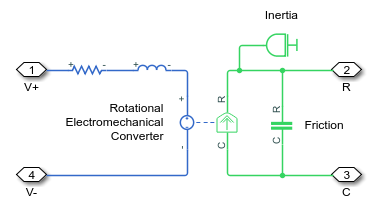## Composite Component — DC Motor

In the Permanent Magnet DC Motor example, the DC Motor block is implemented as a masked subsystem.The following code implements the same model by means of a composite component, called `DC Motor`. The composite component uses the components from the Simscape™ Foundation library as building blocks, and connects them as shown in the preceding block diagram.

```component DC_Motor % DC Motor % This block models a DC motor with an equivalent circuit comprising a % series connection of a resistor, inductor, and electromechanical converter. % Default values are as for the DC Motor Simscape example, ssc_dcmotor. nodes p = foundation.electrical.electrical; % +:left n = foundation.electrical.electrical; % -:left R = foundation.mechanical.rotational.rotational; % R:right C = foundation.mechanical.rotational.rotational; % C:right end parameters rotor_resistance = { 3.9, 'Ohm' }; % Rotor Resistance rotor_inductance = { 12e-6, 'H' }; % Rotor Inductance motor_inertia = { 0.01, 'g*cm^2' }; % Inertia breakaway_torque = { 0.02e-3, 'N*m' }; % Breakaway friction torque coulomb_torque = { 0.02e-3, 'N*m' }; % Coulomb friction torque breakaway_velocity = { 0.1, 'rad/s' }; % Breakaway friction velocity back_emf_constant = { 0.072e-3, 'V/rpm' }; % Back EMF constant end components(ExternalAccess=observe) rotorResistor = foundation.electrical.elements.resistor(R = rotor_resistance); rotorInductor = foundation.electrical.elements.inductor(l = rotor_inductance); rotationalElectroMechConverter = foundation.electrical.elements.rotational_converter(K = ... back_emf_constant); friction = foundation.mechanical.rotational.friction(brkwy_trq = ... breakaway_torque, Col_trq = coulomb_torque, ... brkwy_vel = breakaway_velocity); motorInertia = foundation.mechanical.rotational.inertia(inertia = motor_inertia); end connections connect(p, rotorResistor.p); connect(rotorResistor.n, rotorInductor.p); connect(rotorInductor.n, rotationalElectroMechConverter.p); connect(rotationalElectroMechConverter.n, n); connect(rotationalElectroMechConverter.R, friction.R, motorInertia.I, R); connect(rotationalElectroMechConverter.C, friction.C, C); end end ```

The declaration section of the composite component starts with the `nodes` section, which defines the top-level connection ports of the resulting composite block:

• Two electrical conserving ports, `+` and `-`, on the left side of the block

• Two mechanical rotational conserving ports, `R` and `C`, on the right side of the blockThe `parameters` declaration block lists all the parameters that will be available in the composite block dialog box.The `components` block declares all the member (constituent) components, specifying their complete names starting from the top-level package directory. This example uses the components from the Simscape Foundation library:

• Resistor

• Inductor

• Rotational Electromechanical Converter

• Rotational Friction

• Inertia

The `components` block also links the top-level parameters, declared in the `parameters` declaration block, to the parameters of underlying member components. For example, the Rotor Resistance parameter of the composite block (`rotor_resistance`) corresponds to the Resistance parameter (`R`) of the Resistor block in the Foundation library.

You do not have to link all the parameters of member blocks to top-level parameters. For example, the Rotational Friction block in the Foundation library has the Viscous friction coefficient parameter, which is not mapped to any parameter at the top level. Therefore, the composite model always uses the default value of this parameter specified in the Rotational Friction component, 0.001 N*m/(rad/s).

The `connections` block defines the connections between the nodes (ports) of the member components, and their connections to the top-level ports of the resulting composite block, declared in the `nodes` declaration block of the composite component:

• Positive electrical port `p` of the composite component is connected to the positive electrical port `p` of the Resistor

• Negative electrical port `n` of the Resistor is connected to the positive electrical port `p` of the Inductor

• Negative electrical port `n` of the Inductor is connected to the positive electrical port `p` of the Rotational Electromechanical Converter

• Negative electrical port `n` of the Rotational Electromechanical Converter is connected to the negative electrical port `n` of the composite component

• Mechanical rotational port `R` of the composite component is connected to the following mechanical rotational ports: `R` of the Rotational Electromechanical Converter, `R` of the Rotational Friction, and `I` of the Inertia

• Mechanical rotational port `C` of the composite component is connected to the following mechanical rotational ports: `C` of the Rotational Electromechanical Converter and `C` of the Rotational Friction

These connections are the textual equivalent of the graphical connections in the preceding block diagram.